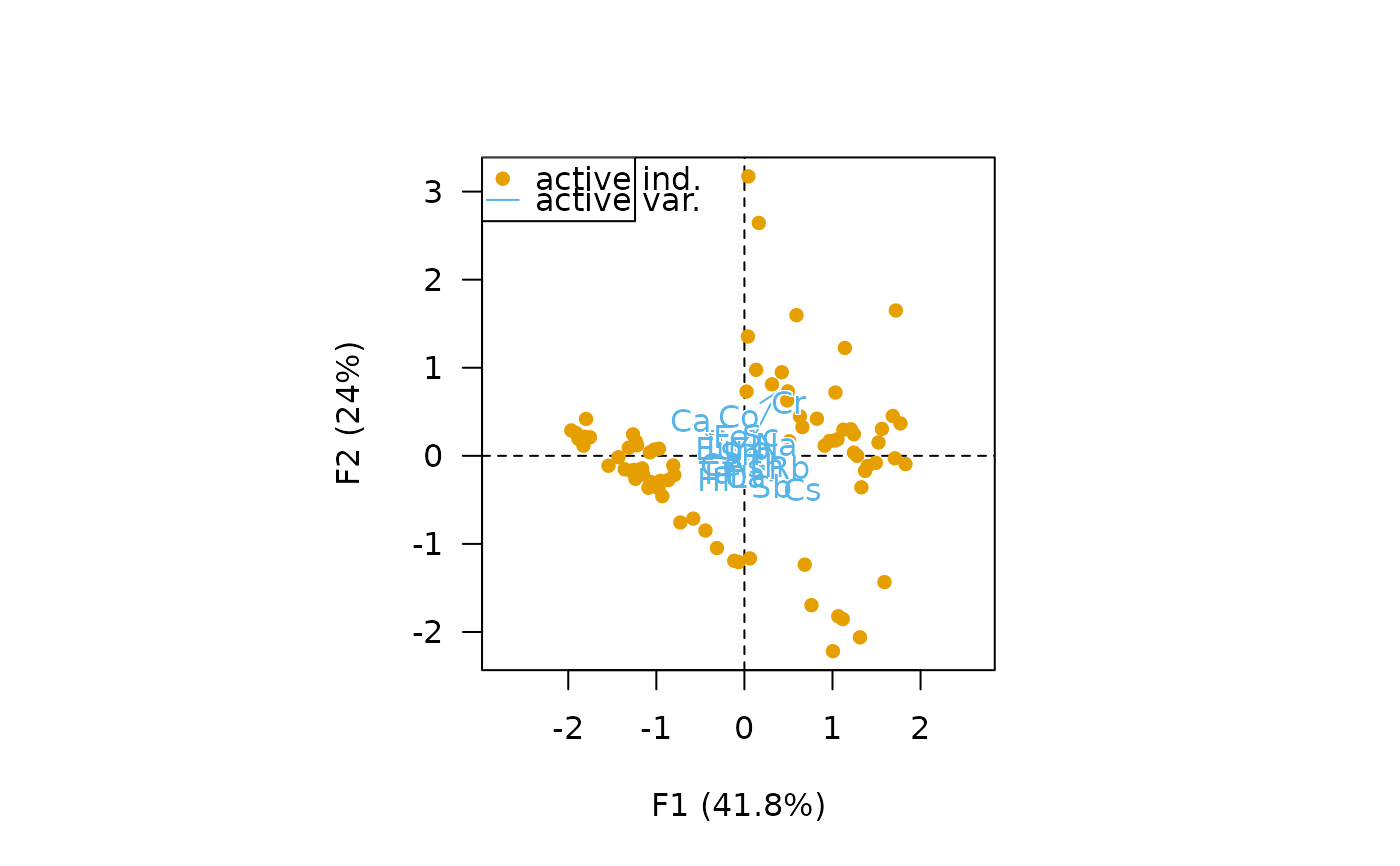Computes a principal components analysis based on the singular value decomposition.

## Usage

# S4 method for CompositionMatrix
pca(
object,
center = TRUE,
scale = TRUE,
rank = NULL,
sup_row = NULL,
sup_col = NULL,
weight_row = NULL,
weight_col = NULL
)

# S4 method for LogRatio
pca(
object,
center = TRUE,
scale = TRUE,
rank = NULL,
sup_row = NULL,
sup_col = NULL,
weight_row = NULL,
weight_col = NULL
)

## Arguments

object

A LogRatio object.

center

A logical scalar: should the variables be shifted to be zero centered?

scale

A logical scalar: should the variables be scaled to unit variance?

rank

An integer value specifying the maximal number of components to be kept in the results. If NULL (the default), $$p - 1$$ components will be returned.

sup_row

A numeric or logical vector specifying the indices of the supplementary rows (individuals).

sup_col

A numeric or logical vector specifying the indices of the supplementary columns (variables).

weight_row

A numeric vector specifying the active row (individual) weights. If NULL (the default), uniform weights are used. Row weights are internally normalized to sum 1

weight_col

A numeric vector specifying the active column (variable) weights. If NULL (the default), uniform weights (1) are used.

## Value

A dimensio::PCA object.

dimensio::pca(), dimensio::biplot()

N. Frerebeau

## Examples

## Coerce to compositional data
data("hongite")
coda <- as_composition(hongite)

## Centered log-ratio
clr <- transform_clr(coda)

## PCA
X <- pca(clr)

## Plot
plot_individuals(X)